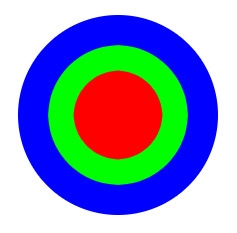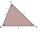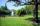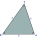# Annulus from triangle

Calculate the content of the area bounded by a circle circumscribed and a circle inscribed by a triangle with sides a = 25mm, b = 29mm, c = 36mm

Correct result:

S =  831 mm2

#### Solution:

$a=25 \ \text{mm} \ \\ b=29 \ \text{mm} \ \\ c=36 \ \text{mm} \ \\ \ \\ c_{1}=\sqrt{ a^2+b^2 }=\sqrt{ 25^2+29^2 } \doteq \sqrt{ 1466 } \ \text{mm} \doteq 38.2884 \ \text{mm} \ \\ c_{1} \ne c \ \\ \ \\ s=\dfrac{ a+b+c }{ 2 }=\dfrac{ 25+29+36 }{ 2 }=45 \ \text{mm} \ \\ A=\sqrt{ s \cdot \ (s-a) \cdot \ (s-b) \cdot \ (s-c) }=\sqrt{ 45 \cdot \ (45-25) \cdot \ (45-29) \cdot \ (45-36) }=360 \ \text{mm}^2 \ \\ \ \\ R=\dfrac{ a \cdot \ b \cdot \ c }{ 4 \cdot \ A }=\dfrac{ 25 \cdot \ 29 \cdot \ 36 }{ 4 \cdot \ 360 }=\dfrac{ 145 }{ 8 }=18.125 \ \text{mm} \ \\ \ \\ r=\dfrac{ A }{ s }=\dfrac{ 360 }{ 45 }=8 \ \text{mm} \ \\ \ \\ S_{1}=\pi \cdot \ R^2=3.1416 \cdot \ 18.125^2 \doteq 1032.0623 \ \text{mm}^2 \ \\ S_{2}=\pi \cdot \ r^2=3.1416 \cdot \ 8^2 \doteq 201.0619 \ \text{mm}^2 \ \\ \ \\ S=S_{1}-S_{2}=1032.0623-201.0619=831 \ \text{mm}^2$Our examples were largely sent or created by pupils and students themselves. Therefore, we would be pleased if you could send us any errors you found, spelling mistakes, or rephasing the example. Thank you!

Leave us a comment of this math problem and its solution (i.e. if it is still somewhat unclear...):Be the first to comment!Tips to related online calculators
Do you want to convert area units?

#### You need to know the following knowledge to solve this word math problem:

We encourage you to watch this tutorial video on this math problem:

## Next similar math problems:

• Sides ratioCalculate the circumference of a triangle with area 84 cm2 if a:b:c = 10:17:21
• Sss triangleCalculate the area and heights in the triangle ABC by sides a = 8cm, b = 11cm, c = 12cm
• Circular railwayThe railway is to interconnect in a circular arc the points A, B, and C, whose distances are | AB | = 30 km, AC = 95 km, BC | = 70 km. How long will the track from A to C?
• Triangular prismCalculate the surface of a triangular prism 10 cm high, the base of which is a triangle with sides 6 cm 8 cm and 8 cm
• Four sides of trapezoidTrapezoid is given by length of four sides: 40.5 42.5 52.8 35.0. Calculate its area.
• Area and two anglesCalculate the size of all sides and internal angles of a triangle ABC, if it is given by area S = 501.9; and two internal angles α = 15°28' and β = 45°.
• Sides of the triangleCalculate triangle sides where its area is S = 84 cm2 and a = x, b = x + 1, xc = x + 2
• Cardboard boxWe want to make a cardboard box shaped quadrangular prism with rhombic base. Rhombus has a side of 5 cm and 8 cm one diagonal long. The height of the box to be 12 cm. The box will be open at the top. How many square centimeters cardboard we need, if we ca
• Trapezoid - hard exampleBase of the trapezoid are: 24, 16 cm. Diagonal 22, 26 cm. Calculate its area and perimeter.
• ParkIn the newly built park will be permanently placed a rotating sprayer irrigation of lawns. Determine the largest radius of the circle which can irrigate by sprayer P so not to spray park visitors on line AB. Distance AB = 55 m, AP = 36 m and BP = 28 m.
• Compute 4Compute the exact value of the area of the triangle with sides 14 mi, 12 mi, and 12 mi long.
• One trapeziumOne trapezium has AB=24M, BC=36M, CD=80M, DA=80M long sides. Find the area.
• Is right triangle or notIf right triangle ABC, have sides a=13, b=11.5, c=22.5. Find area.
• TriangleDetermine whether a triangle can be formed with the given side lengths. If so, use Heron's formula to find the area of the triangle. a = 158 b = 185 c = 201
• Find the areaFind the area of the triangle with the given measurements. Round the solution to the nearest hundredth if necessary. A = 50°, b = 30 ft, c = 14 ft
• The farmerThe farmer would like to first seed his small field. The required amount depends on the seed area. Field has a triangular shape. The farmer had fenced field, so he knows the lengths of the sides: 119, 111 and 90 meters. Find a suitable way to determine t
• SailsWe known heights 220, 165 and 132 of sail. It has triangular shape. What is the surface of the sail?